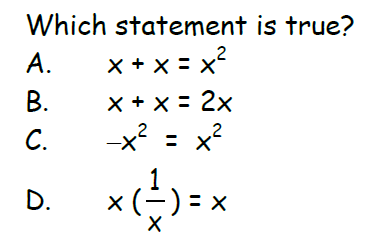Geometry Emergency assignment 1
Problems to sharpen your test skills
Know the definition of complementary *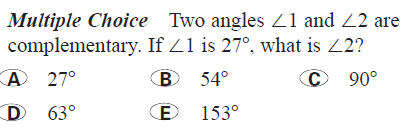Remember what the interior sum of a quadrilateral is?? It's not 180. *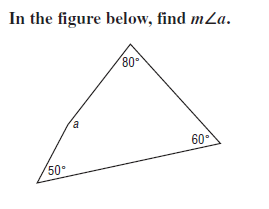Find the rate of change in the temperature per hour. *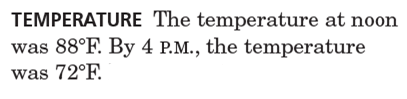This function wants you to plug 6 into x and calculate *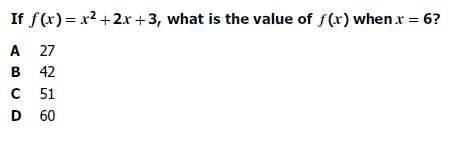This is a perimeter problem. *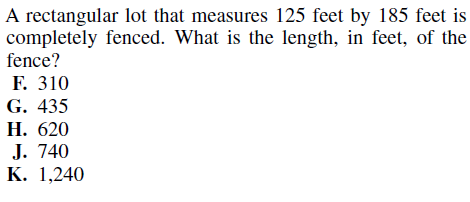Equations *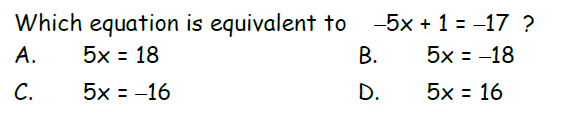Use half circles and vertical angles *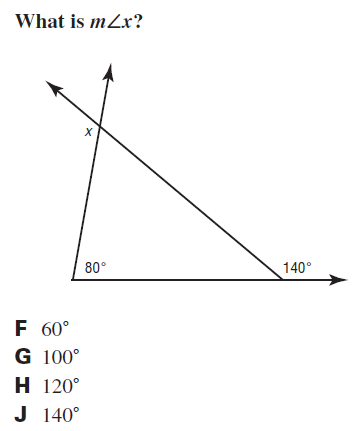Word problem: The cost of renting a row boat is \$40 plus \$50 an hour. How many hours can you row if the total rental fee was \$390 *
Equations *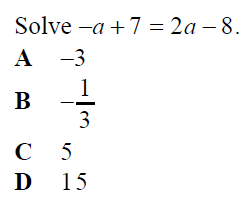Last Name *
If you don't know the midpoint formula, Google it. *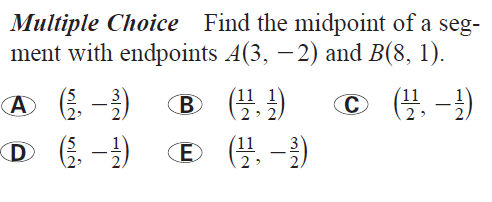period *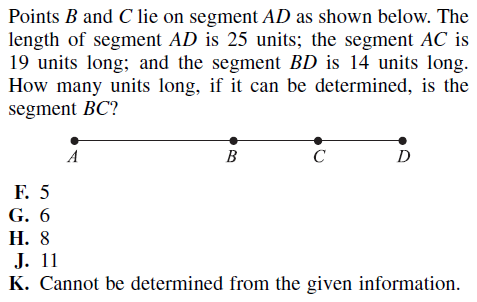Equations *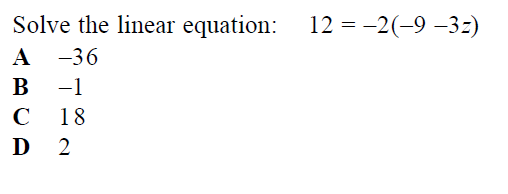Think about what a number needs to be multiplied by in order for the product of the two numbers to equal 1 *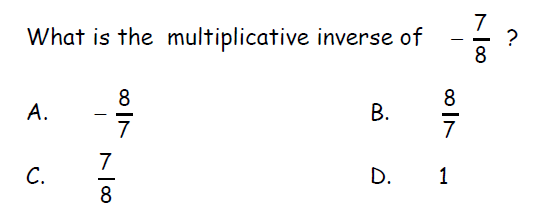Parallel lines have equal slopes and perpendicular lines have opposite reciprocal slopes *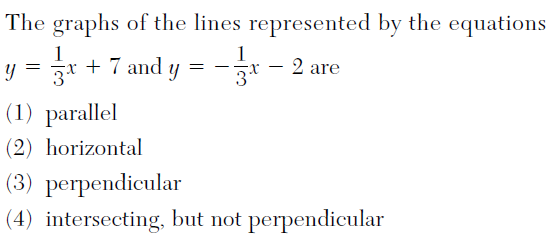Equations *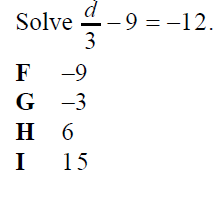arithmetic sequences add and subtract *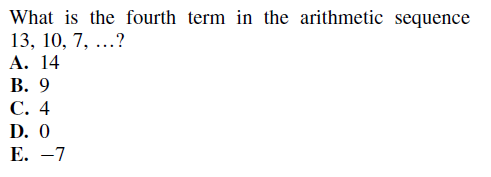Taxes. Remember to add the tax to the total *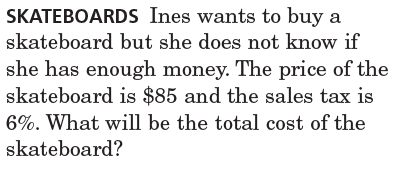Which expression represents the perimeter of this rectangle? *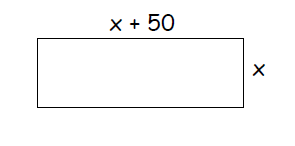Equations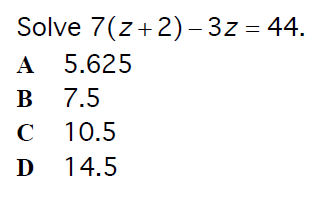Find Rate of change per month for the account. *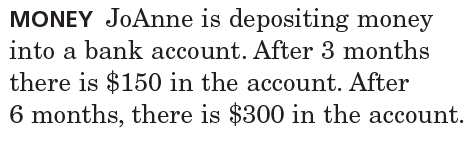Laws of exponents *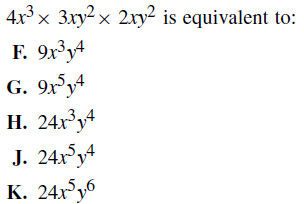*